PHYSICS Mass MASS MA. Fundamental of Mass MB. Kong Frequency and Kong Wavelength MC. Annihilation and Pair Production MD. Kong Equation ME. Kong Atom Model MF. Quantum of Atom MG. Perturbation of Photon MH. Periodic Table MI. Chemical Reaction MJ. Superconductor MK. Particles and Waves ML. Nuclear Physics MB. KONG FREQUENCY AND KONG WAVELENGTH 1. Photon Properties 2. De Broglie Wavelength 3. Kong Frequency and Kong Wavelength 5. Relation of De Broglie Wavelength 6. Property at Low Velocity 7. Davisson-Germer Experiment INTRODUCTION   Electron is particle. As described in chapter "Fundamental of Mass", the arguments which questioned by the author that does electron travel as wave?   Wave is a phenomenon of energy transfer from one point to another point, which does not involve object to move from one point to another point. In particle wave such as water wave and sound wave, particles oscillate between top and bottom, left and right or front and back. Particles oscillate on the same origin and transfer energy without movement from one point to another point. Therefore, moving electrons from one point to another point do not act as particle wave, but comply to the special relativity kinetic energy principle.   As described and shown by Maxwell, electromagnetic (EM) wave is generated through oscillation of electron. If charged particles such as electrons oscillate similarly to water wave when traveling, EM wave will be generated and its energy shall be reducing. But this effect was not observed. Therefore, moving electrons do not act like particle wave.   EM wave travels at the speed of light. Moving particles such as electrons comply to special relativity. As shown by W. Bertozzi for the ultimate speed experiment through accelerating electron, but electrons can never achieve the ultimate speed of light. Therefore, it cannot achieve the properties of EM wave. Particle waves and EM waves are two different properties.   Moving charge particles such as electrons produce magnetic field and respond to external magnetic and electric (M&E) fields. But wave does not respond to external M&E fields.   The diffraction and interference of electrons are described in chapter "Particles and Waves". It is similar to the cathode tube principle where the electrons are diverted due to the M&E field. The diffraction and interference of wave are described in the chapter "Behaviors of Photons". They perform and react the same way upon M&E field.   De Broglie wavelength is commonly used to define the ‘wave’ properties of particles. However, as explained by the author, waves and particles are two separate properties. De Broglie wavelength is not able to describe particles as waves. But it is an alternative terminology to define the energy of particles, in terms of wavelength. This alternative terminology is here called Kong Wavelength.   Here the author derived the related equations of Kong Frequency and Kong Wavelength for moving particles. Chapter ‘Particles and Waves’ describes the reactions of particles in performing the wave reaction. Chapter 'Behaviors of Photons' describes the reactions of photon in performing the wave reaction. Both particle and photon undergoes the same circumstances when producing wave pattern.     OBJECTIVES   1)      To point out the weakness and discrepancy of De Broglie Wavelength 2)      To show that moving particles do not travel as wave. 3)      To derive the Kong Frequency and Kong Wavelength. 4)      To show the relationship between Kong Frequency and Wavelength with EM wave properties. 5)      To show that particles perform and act as wave due to the same kinetic energy level through Davisson- Germer experiment. 6)      To give an example on the Kong Frequency and Kong Wavelength for a moving object. MB.1.0        PHOTON PROPERTIES   The properties of electromagnetic (EM) wave or photon are identified using the followings:- A)    Wavelength B)     Frequency C)    The traveling velocity D)    The energy   For photon, the wavelength is given as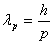… eq. MB.1.1 where   h = Plank’s constant             p = momentum of photon   The traveling velocity of photon is the speed of light, c, where, c = fplp                                                                                                … eq. MB.1.2   The frequency of EM wave is expressed as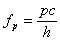… eq. MB.1.3   The energy of photon is expressed as E = hfp                                                                                                … eq. MB.1.4   It is understood that EM wave or photon complies to the above properties and equations. MB.2.0        DE BROGLIE WAVELENGTH   Many physicists accepted that particles possess dual wave-particle aspect. Wave refers to EM wave or photon. It is described that the wavelength of moving particles is given by the De Broglie wavelength. Louis Victor De Broglie stated that the De Broglie wavelength is expressed as,… eq. MB.2.1  where   h = Plank’s constant             p = momentum of moving mass   Eq. MB.2.1 is directly converted from the momentum of photon, which is eq. MB.1.1. But according to the author, the photons and particles are two different properties. The particles travel at speed slower than and can never achieve the speed of light.   The relationship between velocity, frequency and wavelength of traveling particles is v = fl                                                                                                  … eq. MB.2.2   Substitute the De Broglie wavelength, eq. MB.2.1 into eq. MB.2.2, we obtain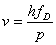… eq. MB.2.3 where   fD = the frequency of moving particle by using De Broglie wavelength   From the special relativity principle, the momentum of moving particle is p = gmv                                                                                               … eq. MB.2.4 where   g = Lorentz factor             m = mass of moving particle             v = velocity of moving particle   Substitute eq. MB.2.4 into eq. MB.2.3, we obtain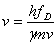… eq. MB.2.5   Rearrange eq. MB.2.5 to obtain the energy and frequency of moving particle using De Broglie Wavelength, we have ED = hfD = gmv2                                                                                 … eq. MB.2.6… eq. MB.2.7   Eq. MB.2.6 is not correct for all levels of velocity of moving objects. It is not comply to special relativity principle. And it is not tally with eq. MB.1.4. This is one of the discrepancies for De Broglie’s suggestion.   Eq. MB.2.7 is not tally with eq. MB.1.3, where eq. MB.1.3 shows the frequency property of photon. Once again, De Broglie’s suggestion failed.   In order to show that particles travel as photon, eq. MB.2.2 must tally with eq. MB.1.2, eq. MB.2.6 must tally with eq. MB.1.4 and eq. MB.2.7 must tally with eq. MB.1.3. The findings are although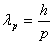is tally with… eq. MB.2.8   But, c = fplp is not tally with v = fDlD                                                        … eq. MB.2.9 E = hfp is not tally with ED = hfD                                                         … eq. MB.2.10is not tally with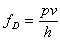… eq. MB.2.11   From the above, it is shows that De Broglie wavelength fails to show that moving particles travel as photon. In short, we can conclude that moving particles do not travel as EM wave or photon. MB.3.0        KONG FREQUENCY AND KONG WAVELENGTH   As mentioned above, De Broglie wavelength is not able to describe that moving particle is photon. But it is an alternative terminology to define the energy of particles. The moving particles can be expressed in terms of frequency and wavelength. In this chapter, we develop the properties of moving particles and show the relationship between photons and moving particles.   For moving particles, the velocity of moving particle can be expressed in terms of frequency and wavelength as follows:-   v = fKlK                                                                                              …eq. MB.3.1 where   fK = frequency of moving particle = The Kong Frequency             lK = wavelength of moving particle = The Kong Wavelength     MB.3.1        Kong Frequency   We begin the derivation by using the special relativity Grand Total Energy equation as follow,   E2 = (pc)2 + (mc2)2                                                                              … eq. MB.3.2 where   E = total energy of system p = momentum of moving particles             m = mass of moving particles             c = speed of light   Eq. MB.3.2 can be expressed graphically as shown in figure MB.3.1 below.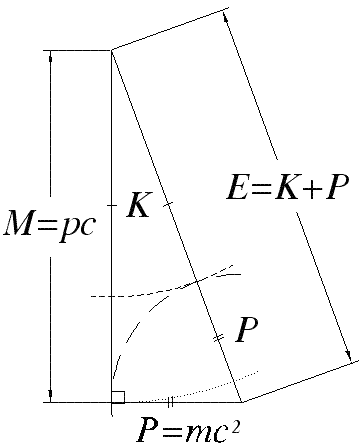Figure MB.3.1. K is the kinetic energy of moving particle. P is the potential energy of moving particle. M is related to the momentum of moving particle. E, M and P are trigonometric related and expressed by eq. MB.3.2.   From special relativity principle, the total system energy of moving particle is given as, E = gmc2 = K + P                                                                              … eq. MB.3.3 where   g = Lorentz factor             m = mass of moving particle             c = speed of light   The total energy can be expressed in term of frequency as follow, E = gmc2 = hfK                                                                                   … eq. MB.3.4 where   h = Plank’s constant             fK = the Kong Frequency   Rearrange eq. MB.3.4 to obtain the Kong Frequency, we have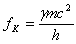… eq. MB.3.5     MB.3.2        Kong Wavelength   Substitute eq. MB.3.5 into eq. MB.3.1 and rearrange, we obtain the Kong Wavelength,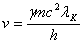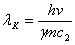… eq. MB.3.6   Eq. MB.3.4 is the total energy of moving particle. Eq. MB.3.5 is the Kong Frequency and eq. MB.3.6 is the Kong wavelength MB.4.0        RELATION BETWEEN PHOTON AND MOVING PARTICLE   From figure MB.3.1, for photon model, the mass of photon is zero. Therefore the second term of the right side of eq. MB.3.2 is zero. The total energy for photon system is,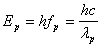… eq. MB.4.1 where   h = Plank’s constant             fp = frequency of photon             lp = wavelength of photon   The energy of photon is the component ‘M’ of figure MB.3.1. At this photon energy level, the photon undergoes wave reactions such as diffraction at the wavelength of lp. In order for the moving particle to undergo the same reaction, the resultant energy level of moving particle must be equivalent to the same energy level of the photon. The component ‘M’ of moving particle must possess the equivalent photon energy level.   We first find the relation between the Kong Frequency and Wavelength with the photon frequency and wavelength.     MB.4.1        Relation Between Kong Frequency and Photon Frequency   From the grand total energy equation, E2 = M2 + P2                                                                                        … eq. MB.4.2   In order to perform the same reaction, we substitute the component ‘M’ with eq. MB.4.1, we obtain, (gmc2)2 = (hfp)2 + (mc2)2                                                                     … eq. MB.4.3 (hfp)2 = (g2-1)(mc2)2                                                                             … eq. MB.4.4 (hfp)2 = (gb)2(mc2)2                                                                              … eq. MB.4.5 where   (g2-1) = (gb)2             g = Lorentz factor             b = speed parameter of special relativity   Square-root both side of eq. MB.4.5, we obtain, hfp = gbmc2                                                                                         … eq. MB.4.6   Substitute eq. MB.3.4 into eq. MB.4.6, we obtain, hfp = bhfK                                                                                           … eq. MB.4.7   Rearrange eq. MB.4.7, we obtain the relation between the Kong Frequency and the photon frequency is fp = bfK                                                                                               … eq. MB.4.8     MB.4.2        Relation Between Kong Wavelength and Photon Wavelength   Substitute eq. MB.1.2 and MB.3.1 into eq. MB.4.8, we obtain,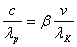… eq. MB.4.9   Rearrange eq. MB.4.9, we obtain, lK = b2lp                                                                                            … eq. MB.4.10   Eq. MB.4.10 shows the relation between the Kong Wavelength and the photon wavelength.   In order for the moving particles to diffract according to the photon wavelength of lp, the moving particles must possess the Kong Wavelength of lK, adhere to the relation of eq. MB.4.10.   In order for the moving particles to diffract according to the photon wavelength of lp, the moving particles must possess the Kong Frequency of fK, adhere to the relation of eq. MB.4.8 with the photon frequency.   The Kong Frequency and the Kong Wavelength are the alternative terminologies to describe the energy level of moving particle.   We can then summarize the relations in the figure MB.4.1 below.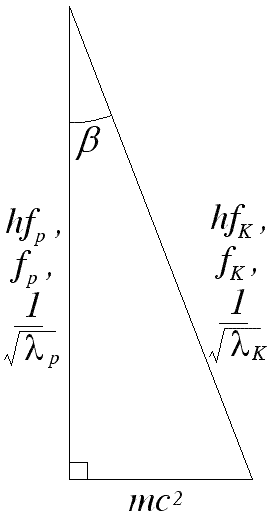Figure MB.4.1     MB.4.3        Relation Between Photon Energy and Kinetic Energy   From eq. MB.4.6, we substitute the special relativity kinetic energy equation into eq. MB.4.6, we obtain the relation between the photon energy and the kinetic energy of moving particles,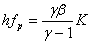… eq. MB.4.11 where   K = (g-1)mc2   Eq. MB.4.9 shows the relation of component ‘M’.   Substitute eq. MB.1.2 into eq. MB.4.11, we obtain,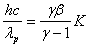… eq. MB.4.12   Rearrange eq. MB.4.12, we obtain,… eq. MB.4.13   In order for the moving particle to diffract with photon wavelength, lp, the moving particle shall possess the kinetic energy of K. The special relativity kinetic energy can be expressed in Electron-Volt as follow, K = eV                                                                                                … eq. MB.4.14 where   e = electron charge             V = accelerating potential MB.5.0        RELATION OF DE BROGLIE WAVELENGTH   The Kong Wavelength represents the wavelength of moving particles in relation to the energy level of moving particles. In this chapter, we find out the relation between the Kong wavelength and the De Broglie wavelength.   From eq. MB.3.4, we have, hfK = gmc2                                                                                           … eq. MB.5.1   Substitute eq. MB.3.1 into eq. MB.5.1, we obtain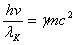… eq. MB.5.2   Rearrange to obtain the Kong Wavelength,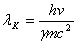… eq. MB.5.3   From eq, MB.4.10, the diffracted wavelength is,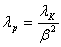… eq. MB.5.4   Substitute eq. MB.5.3 into eq. MB.5.4, we obtain,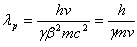… eq. MB.5.5   Substitute eq. MB.2.4 into eq. MB.5.5, we obtain,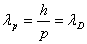… eq. MB.5.6 where   lD = the De Broglie wavelength.   The De Broglie wavelength represents the diffracted wavelength of moving particles due to the same energy level between moving particles and photons. MB.6.0        PROPERTIES AT LOW VELOCITY   From equation MB.3.4, the total energy is hfK = gmc2                                                                                           … eq. MB.6.1   For particle travels at low velocity, the total energy becomes hfK = (1-b2)-1/2 mc2                                                                              ... eq. MB.6.2 where   g = (1-b2)-1/2   We then expand the Lorentz factor and the speed parameter using binomial theorem as follows, g = 1 + ½b2 – ¼b4 + 1/8b8 – …                                                           … eq. MB.6.3   When v << c, the speed parameter is small. We can then, with litter error, ignore the higher power term of the speed parameter and remain the first two terms. We then obtain, g » 1 + ½b2                                                                                         … eq. MB.6.4   Substitute eq. MB .6.4 into eq. MB.6.2, we obtain hfK = (1 + ½b2)mc2       = ½mv2 + mc2            = eV + mc2       = K + P                                                                                        … eq. MB.6.5   Eq. MB.6.5 is the total energy of moving particle at low velocity. The Kong Frequency at low velocity is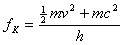… eq. MB.6.6   Substitute eq. MB.3.1 into eq. MB.6.6, we obtain the Kong wavelength at low velocity,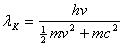… eq. MB.6.7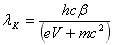… eq. MB.6.8 MB.7.0        DAVISSON-GERMER EXPERIMENT   In 1937, Davisson and Germer shared the Nobel Prize in Physics for their work in measuring the wavelength of electron at low velocity. Electron incident beam is shot on nickel crystal and the angle of diffracted beam is measured. The diffracted beam is formed by Bragg reflection of the electron from a particular family of atomic planes within the crystal. When the parallel diffraction is obtained, the first-order diffraction peak is measured and the diffraction angle measured is 50°. The calculated wavelength using first-order diffraction equation is 165 pm. The energy of electron incident beam is 54 eV.   The kinetic energy is 54 eV and the speed parameter of electron incident beam, b = 0.014538. Substitute into eq. MB.6.8, the Kong wavelength is,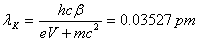… eq. MB.7.1   The diffracted wavelength is given by eq. MB.4.10,… eq. MB.7.2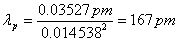The calculated result is in good agreement with the measured value with 1% experiment error. The same result is obtained when De Broglie wavelength is applied.   The Kong Frequency of the electron incident beam is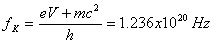… eq. MB.7.3   The equivalent photon frequency given by eq. MB.4.8 is fp = bfK = 1.80x1018 Hz                                                                      … eq. MB.7.4   The velocity of the electron incident beam is v = bc = 4.358x106 m/s                                                                      … eq. MB.7.5 MB.8.0        PROPERTIES OF A MOVING BASEBALL   A baseball with mass 150g travels at speed 35.0m/s. The expressions of the moving object in terms of frequency and wavelength are   The Kong Frequency is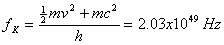… eq. MB.8.1   The Kong wavelength is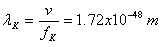… eq. MB.8.2   The total energy is E = hfK = 1.35x1016 J                                                                         … eq. MB.8.3   If the baseball undergoes diffraction, the diffracted wavelength is… eq. MB.8.4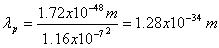… eq. MB.8.5   The equivalent photon frequency given by eq. MB.4.8 is fp = bfK = 2.35x1042 Hz                                                                      … eq. MB.8.6   Eq. MB.8.1 and eq. MB.8.2 shows the properties of the baseball in term of frequency and wavelength. But it does not mean that the basebasll is traveling as wave.   Eq. MB.8.5 and eq. MB.8.6 shows the properties of the baseball if it is diffracted. Again, it does not mean that the baseball is traveling as wave. DISCUSSIONS AND CONCLUSIONS   The properties of EM wave or photon consist of four aspects. There are the wavelength, frequency, traveling speed and total energy of photon. For a substance to be categorized as photon, it must comply to all the four aspects.   The suggestion by De Broglie only complies to one aspect but not comply to the other 3 aspects. Therefore, the suggestion made is inadequate to conclude that the moving particle is traveling as wave. In fact, the moving particle is not traveling as wave. It adheres to the special relativity principle.   The energy of photon is the component ‘M’ of figure MB.3.1. At this photon energy level, the photon undergoes wave reactions such as diffraction at the wavelength of lp. In order for the moving particle to undergo the same reaction, the resultant energy level of moving particle must be equivalent to the same energy level of the photon. The component ‘M’ of moving particle must possess the equivalent photon energy level.   Eq. MB.4.7 and eq. MB.4.11 shows the relation between photon energy and moving particle energy who undergoes the same diffraction pattern.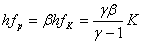… eq. MB.9.1   The photon energy and the kinetic energy of moving particles are related according to eq. MB.9.1. It is the relation of the component ‘M’ of figure MB.3.1. Figure MB.4.1 shows the graphical relation between the properties of photon and moving particle.   Left side of eq. MB.9.1 represents the photon energy, while the right side represents the kinetic energy of moving object. The middle is the relation of the Kong Frequency to the equivalent photon energy.   Photon travels at the speed of light, while moving particles travel at speed lower than speed of light. Both photon energy and kinetic energy of moving particles are related according to eq. MB.9.1.   For photons and particles whose possess the same energy level, they perform and react the same way upon the same M&E field. Therefore, the diffraction pattern produced by waves and particles incident beam is the same as shown in Davisson-Germer experiment.   Again, we state that waves, regardless of particle or EM waves, is one kind of energy transfer from one point to another point without displacement of particles. Moving particles from one point to another point is not complying to the characteristic of wave.   Oscillation of charged particles such as electrons, produce EM wave, but this is not observed in electron incident beam. Therefore, moving electrons do not oscillate and do not travel in the similar way of particle wave.   Moving charged particles produce circular magnetic field, but traveling EM waves and particle waves do not produce magnetic field. Therefore, electron incident beam does not travel as wave.   Chapter ‘Particles and Waves’ describes the reactions of particles in performing the wave characteristic. It is due to the Kong Effect where the electrons incident beam reacts upon the M&E field. This phenomenon is similar to the principle of cathode tube.   The topic “Wave and Photon” describes the reactions of photon in performing the wave characteristic. Both particle and photon undergoes the same circumstances when producing wave pattern.   Since moving particles do not travel as wave, why the electrons do not collapse into the nucleus of atom? This is discussed from chapter ‘Kong Equation’ onwards.

This website is originated on 15-Mar-2007,

updated on 4-Jan-2009.